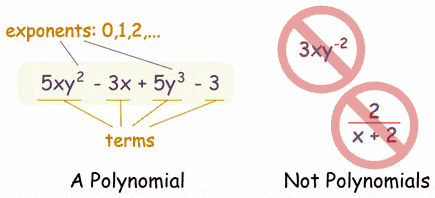# Expression, Terms, Factors, Coefficients, Mono/Bi/Poly HELP

• I
kyphysics
I'm trying to clarify my terminological understanding of things related to mathematical expressions.

Actually, first I want to make sure I understand what an expression is and is not.

From my current understanding, an expression is any number, variable, or combination of numbers and variables linked together by addition or subtraction.

Examples:
4 + 3
4x +3
1/3x^2 - 14x + 2y

The components of an expression are:
terms, factors, constants, and coefficients

Terms are the values linked by +/-. Factors are the things being multiplied (e.g., 4 and x in the second example above). Constants are numbers (like the 3 in first and second example). And coefficients are the numbers in front of variables (like 4 and 1/3 in the second and third example above. Am I right about this so far?

Also, in the third example, the second term is 14x. Would we say it's 14x or -14x. Similarly, would we say the coefficient is 14 or -14?

Questions:
What are monomials, binomials, and polynomials? Are they simply types of expressions?

On the "Math Is Fun" website, they state:
A polynomial can have constants, variables and the exponents 0,1,2,3,...

But it never has division by a variable.

I'm slightly confused.

1.) So, polynomials can't have variables in the denominator, but they can have a denominator?
2.) Also, they can't have negative exponents, but they can have exponents?
3.) I've seen on a YouTube video a person saying that monomials, binomials, trinomials, etc. are ALL polynomials, because "poly" means many and doesn't specify the number of terms. So, technically, according to this YouTube video maker, monomials are also polynomials. Is this true?

Just trying to nail down my terminology!The above image was taken from: https://www.mathsisfun.com/algebra/definitions.html

## Answers and Replies

Homework Helper
I think of an expression as a general term for any mathematical phrase. e.g. ##\sqrt{x^{1.3}-\log{y}}## or ##x^2 ##.
Also, in the third example, the second term is 14x. Would we say it's 14x or -14x. Similarly, would we say the coefficient is 14 or -14?
I would recommend keeping the negative sign with your coefficient. This way, you can generally refer to the expression as a sum of terms.
What are monomials, binomials, and polynomials? Are they simply types of expressions?
Yes. Specifically, monomials and binomials are specific categories of polynomials.
Monomials will have the form:
##a x^\alpha## where ## a\in \mathbb{R} \neq 0## and ##\alpha \in \mathbb{Z} \geq 0.##
Similarly binomials will have exactly two terms.
##a x^\alpha + bx^\beta## where ## a,b \in \mathbb{R} \neq 0## and ##\alpha, \beta \in \mathbb{Z} \geq 0, \alpha \neq \beta.##
1.) So, polynomials can't have variables in the denominator, but they can have a denominator?
Right, but the denominator is a constant, and is usually thought of as part of the coefficient.
i.e. ## \frac{x^2}{2}##
2.) Also, they can't have negative exponents, but they can have exponents?
Right. To be a polynomial, you are only allowed to have non-negative integer exponents on your variable.
3.) I've seen on a YouTube video a person saying that monomials, binomials, trinomials, etc. are ALL polynomials, because "poly" means many and doesn't specify the number of terms. So, technically, according to this YouTube video maker, monomials are also polynomials. Is this true?
Yes.